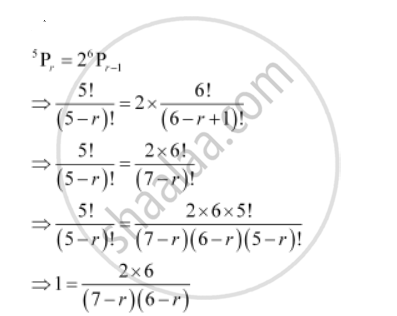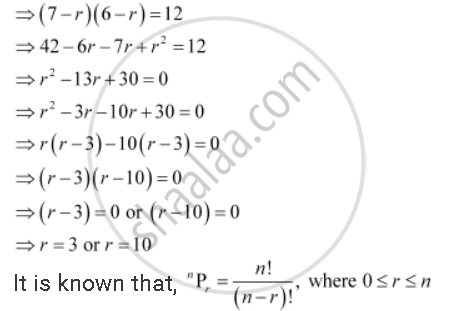Share

# Find R If 5p_R = 2^6 P_(R-1) Permutations, - Mathematics

#### Question

Find r if ""^5P_r = 2^6 P_(r-1)

#### Solution∴0 ≤ ≤ 5

Hence, r ≠ 10

r = 3

Is there an error in this question or solution?